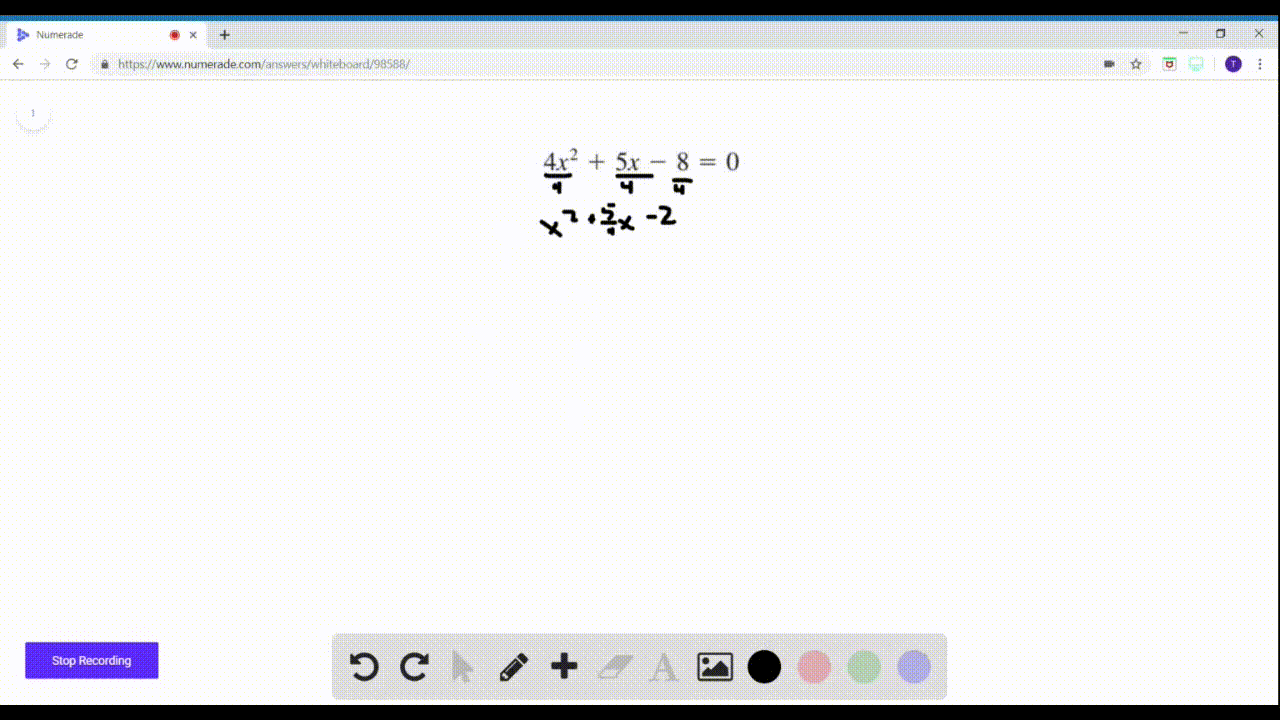Enroll in one of our FREE online STEM summer camps. Space is limited so join now!View Summer Courses### $17-28=$ Completing the Square Find all real solu…

03:01Central Michigan University

Need more help? Fill out this quick form to get professional live tutoring.

Get live tutoring
Problem 27

$17-28=$ Completing the Square Find all real solutions of the
equation by completing the square.
$$2 x^{2}+7 x+4=0$$

$x=-\frac{7}{4} \pm \frac{\sqrt{17}}{4}$

## Discussion

You must be signed in to discuss.

## Video Transcript

all right. Fun stuff here with the solving quadratic equations. By completing the square, you're getting a little bit more advanced, a little bit more difficult. If I wasn't being told to solve this with completing the square, it probably used the quadratic formula. But here we go. So let's start by isolating the X squared term and the ex term, Um, by subtracting four from both sides, that's the first thing we gotta D'oh. Then I'm gonna divide everything by two to get the, um, lead coefficient to be one. Then I can actually do what it's called quote unquote completing the square. So what do I add to this left side? To make it a perfect square, try no meal. You take half of the B value half of seven houses, seven force and then you square it. 74 squared is 49 over 16 seven scores 49 4 square to 16. So we're going to add 49 16th to both side. That's called completing the Square. Um, I am gonna change negative to two negative. 32 16th. That's just getting myself a common denominator, because I'm gonna have to add those anyway, so Why don't we add 49 16 support side? Why did we take half of this? Be value and square it and and the left side so we could make it a perfect square trey. No meal that can be factored easily as this. It would be X squared on that X squared, but acts plus seven hats, seven forced squared. So that's the factory ization of this. All right, on the right, we simplify, and we get 17 16th. Then we can take thes square root herbal sides. Um, and we get X plus seven. Force equals plus or minus the swirl of 17 over for. So the square to 17 is the square to 17. Scored. 16 is four. We're just one step away from heaviness. All factor is gonna give myself a little bit more room here. I have an assault, brother, and that is to subtract seven force from both sides. Negative seven. Force closer Money. Nous the square root of 17 over four. That is our solution. It is not rational, but and it's ugly, but it's our answer number less negative seven force plus or minus two squared of 17 force